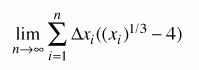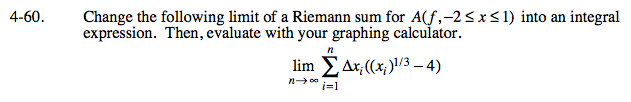### Home > CALC > Chapter 4 > Lesson 4.2.2 > Problem4-60

4-60.
1. Change the following limit of a Riemann sum for A(f, −2 ≤ x ≤ 1) into an integral expression. Then, evaluate with your graphing calculator. Homework Help ✎The Definition of an Integral is

Can you rewrite the given Riemann sum as an integral?

It was given that a = −2 and b = 1.